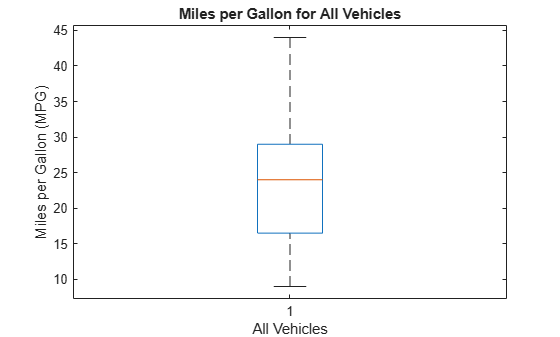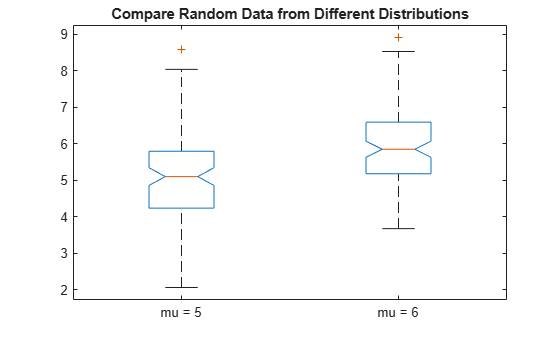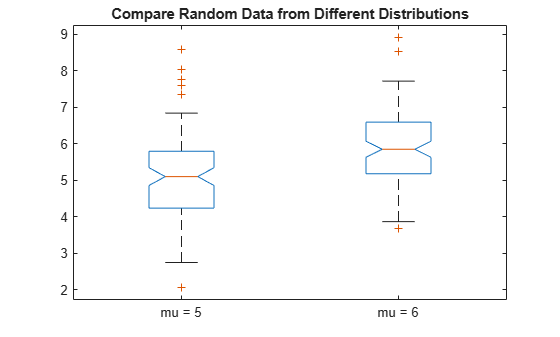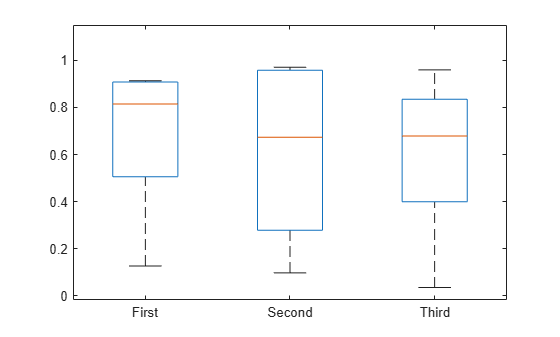# boxplot

Visualize summary statistics with box plot

## Syntax

``boxplot(x)``
``boxplot(x,g)``
``boxplot(ax,___)``
``boxplot(___,Name,Value)``

## Description

example

````boxplot(x)` creates a box plot of the data in `x`. If `x` is a vector, `boxplot` plots one box. If `x` is a matrix, `boxplot` plots one box for each column of `x`. On each box, the central mark indicates the median, and the bottom and top edges of the box indicate the 25th and 75th percentiles, respectively. The whiskers extend to the most extreme data points not considered outliers, and the outliers are plotted individually using the `'+'` marker symbol.```

example

````boxplot(x,g)` creates a box plot using one or more grouping variables contained in `g`. `boxplot` produces a separate box for each set of `x` values that share the same `g` value or values.```
````boxplot(ax,___)` creates a box plot using the axes specified by the axes graphic object `ax`, using any of the previous syntaxes.```

example

````boxplot(___,Name,Value)` creates a box plot with additional options specified by one or more `Name,Value` pair arguments. For example, you can specify the box style or order.```

## Examples

collapse all

`load carsmall`

Create a box plot of the miles per gallon (`MPG`) measurements. Add a title and label the axes.

```boxplot(MPG) xlabel('All Vehicles') ylabel('Miles per Gallon (MPG)') title('Miles per Gallon for All Vehicles')```The boxplot shows that the median miles per gallon for all vehicles in the sample data is approximately 24. The minimum value is about 9, and the maximum value is about 44.

`load carsmall`

Create a box plot of the miles per gallon (`MPG`) measurements from the sample data, grouped by the vehicles' country of origin (`Origin`). Add a title and label the axes.

```boxplot(MPG,Origin) title('Miles per Gallon by Vehicle Origin') xlabel('Country of Origin') ylabel('Miles per Gallon (MPG)')```Each box visually represents the MPG data for cars from the specified country. Italy's "box" appears as a single line because the sample data contains only one observation for this group.

Generate two sets of sample data. The first sample, `x1`, contains random numbers generated from a normal distribution with `mu = 5` and `sigma = 1`. The second sample, `x2`, contains random numbers generated from a normal distribution with `mu = 6` and `sigma = 1`.

```rng default % For reproducibility x1 = normrnd(5,1,100,1); x2 = normrnd(6,1,100,1);```

Create notched box plots of `x1` and `x2`. Label each box with its corresponding `mu` value.

```figure boxplot([x1,x2],'Notch','on','Labels',{'mu = 5','mu = 6'}) title('Compare Random Data from Different Distributions')```The boxplot shows that the difference between the medians of the two groups is approximately 1. Since the notches in the box plot do not overlap, you can conclude, with 95% confidence, that the true medians do differ.

The following figure shows the box plot for the same data with the maximum whisker length specified as 1.0 times the interquartile range. Data points beyond the whiskers are displayed using `+`.

```figure boxplot([x1,x2],'Notch','on','Labels',{'mu = 5','mu = 6'},'Whisker',1) title('Compare Random Data from Different Distributions')```With the smaller whiskers, `boxplot` displays more data points as outliers.

Create a 100-by-25 matrix of random numbers generated from a standard normal distribution to use as sample data.

```rng default % For reproducibility x = randn(100,25);```

Create two box plots for the data in `x` on the same figure. Use the default formatting for the top plot, and compact formatting for the bottom plot.

```figure subplot(2,1,1) boxplot(x) subplot(2,1,2) boxplot(x,'PlotStyle','compact')```Each plot presents the same data, but the compact formatting may improve readability for plots with many boxes.

Create box plots for data vectors of varying length by using a grouping variable.

Randomly generate three column vectors of varying length: one of length `5`, one of length `10`, and one of length `15`. Combine the data into a single column vector of length `30`.

```rng('default') % For reproducibility x1 = rand(5,1); x2 = rand(10,1); x3 = rand(15,1); x = [x1; x2; x3];```

Create a grouping variable that assigns the same value to rows that correspond to the same vector in `x`. For example, the first five rows of `g` have the same value, `First`, because the first five rows of `x` all come from the same vector, `x1`.

```g1 = repmat({'First'},5,1); g2 = repmat({'Second'},10,1); g3 = repmat({'Third'},15,1); g = [g1; g2; g3];```

Create the box plots.

`boxplot(x,g)`## Input Arguments

collapse all

Input data, specified as a numeric vector or numeric matrix. If `x` is a vector, `boxplot` plots one box. If `x` is a matrix, `boxplot` plots one box for each column of `x`.

On each box, the central mark indicates the median, and the bottom and top edges of the box indicate the 25th and 75th percentiles, respectively. The whiskers extend to the most extreme data points not considered outliers, and the outliers are plotted individually using the `'+'` marker symbol.

Data Types: `single` | `double`

Grouping variables, specified as a numeric vector, character array, string array, cell array, or categorical array. You can specify multiple grouping variables in `g` by using a cell array of these variable types or a matrix. If you specify multiple grouping variables, they must all be the same length.

If `x` is a vector, then the grouping variables must contain one row for each element of `x`. If `x` is a matrix, then the grouping variables must contain one row for each column of `x`. Groups that contain a missing value (`NaN`), an empty character vector, an empty or `<missing>` string, or an `<undefined>` value in a grouping variable are omitted, and are not counted in the number of groups considered by other parameters.

By default, `boxplot` sorts character and string grouping variables in the order they initially appear in the data, categorical grouping variables by the order of their levels, and numeric grouping variables in numeric order. To control the order of groups, do one of the following:

• Use categorical variables in `g` and specify the order of their levels.

• Use the `'GroupOrder'` name-value pair argument.

Data Types: `single` | `double` | `char` | `string` | `cell` | `categorical`

Axes on which to plot, specified as an axes graphic object. If you do not specify `ax`, then `boxplot` creates the plot using the current axis. For more information on creating an axes graphic object, see `axes` and Axes Properties.

### Name-Value Arguments

Specify optional pairs of arguments as `Name1=Value1,...,NameN=ValueN`, where `Name` is the argument name and `Value` is the corresponding value. Name-value arguments must appear after other arguments, but the order of the pairs does not matter.

Before R2021a, use commas to separate each name and value, and enclose `Name` in quotes.

Example: `'Notch','on','Labels',{'mu = 5','mu = 6'}` creates a notched box plot and labels the two boxes `mu = 5` and ```mu = 6```, from left to right

Box Appearance

collapse all

Box style, specified as one of the following.

NameValue
`'outline'`Plot boxes using an unfilled box with dashed whiskers. This is the default if `'PlotStyle'` is `'traditional'`.
`'filled'`Plot boxes using a narrow filled box with lines for whiskers. This is the default if `'PlotStyle'` is `'compact'`.

Example: `'BoxStyle','filled'`

Box colors, specified as an RGB triplet, character vector, or string scalar. An RGB triplet is a three-element row vector whose elements specify the intensities of the red, green, and blue components of the color, respectively. Each intensity must be in the range [0,1].

The following table lists the available color characters and their equivalent RGB triplet values.

Long NameShort NameRGB Triplet
Yellow`'y'``[1 1 0]`
Magenta`'m'``[1 0 1]`
Cyan`'c'``[0 1 1]`
Red`'r'``[1 0 0]`
Green`'g'``[0 1 0]`
Blue`'b'``[0 0 1]`
White`'w'``[1 1 1]`
Black`'k'``[0 0 0]`

You can specify multiple colors either as a character vector or string scalar of color names (for example, `'rgbm'`) or a three-column matrix of RGB values. The sequence is replicated or truncated as required, so for example, `'rb'` gives boxes that alternate red and blue.

If you do not specify the name-value pair `'ColorGroup'`, then `boxplot` uses the same color scheme for all boxes. If you do specify `'ColorGroup'`, then the default is a modified `hsv` `colormap`.

Example: `'Colors','rgbm'`

Median style, specified as one of the following.

NameValue
`'line'`Draw a line to represent the median in each box. This is the default when `'PlotStyle'` is `'traditional'`.
`'target'`Draw a black dot inside a white circle to represent the median in each box. This is the default when `'PlotStyle'` is `'compact'`.

Example: `'MedianStyle','target'`

Marker for comparison intervals, specified as one of the following.

NameValue
`'off'`Omit comparison intervals from box display.
`'on'`If `'PlotStyle'` is `'traditional'`, draw comparison intervals using notches. If `'PlotStyle'` is `'compact'`, draw comparison intervals using triangular markers.
`'marker'`Draw comparison intervals using triangular markers.

Two medians are significantly different at the 5% significance level if their intervals do not overlap. `boxplot` represents interval endpoints using the extremes of the notches or the centers of the triangular markers. The notch extremes correspond to q2 – 1.57(q3q1)/sqrt(n) and q2 + 1.57(q3q1)/sqrt(n), where q2 is the median (50th percentile), q1 and q3 are the 25th and 75th percentiles, respectively, and n is the number of observations without any `NaN` values. If the sample size is small, the notches might extend beyond the end of the box.

Example: `'Notch','on'`

Marker size for outliers, specified as a positive numeric value. The specified value represents the marker size in points.

If `'PlotStyle'` is `'traditional'`, then the default value for `OutlierSize` is 6. If `'PlotStyle'` is `'compact'`, then the default value for `OutlierSize` is 4.

Example: `'OutlierSize',8`

Data Types: `single` | `double`

Plot style, specified as one of the following.

NameValue
`'traditional'`Plot boxes using a traditional box style.
`'compact'`Plot boxes using a smaller box style designed for plots with many groups. This style changes the defaults for some other parameters.

Example: `'PlotStyle','compact'`

Marker and color for outliers, specified as a character vector or string scalar containing symbols for the marker and color. The symbols can appear in any order. If you omit the marker symbol, then outliers are invisible. If you omit the color symbol, then outliers appear in the same color as the box.

If `'PlotStyle'` is `'traditional'`, then the default value is `'+r'`, which plots each outlier using a red plus sign `'+'` marker symbol.

If `'PlotStyle'` is `'compact'`, then the default value is `'o'`, which plots each outlier using a circle `'o'` marker symbol in the same color as the corresponding box.

MarkerDescriptionResulting Marker
`'o'`Circle`'+'`Plus sign`'*'`Asterisk`'.'`Point`'x'`Cross`'_'`Horizontal line`'|'`Vertical line`'s'`Square`'d'`Diamond`'^'`Upward-pointing triangle`'v'`Downward-pointing triangle`'>'`Right-pointing triangle`'<'`Left-pointing triangle`'p'`Pentagram`'h'`HexagramColorDescription

`'y'`

Yellow

`'m'`

Magenta

`'c'`

Cyan

`'r'`

Red

`'g'`

Green

`'b'`

Blue

`'w'`

White

`'k'`

Black

Example: Specify `'Symbol',''` to make the outliers invisible.

Box width, specified as a numeric scalar or numeric vector. If the number of boxes is not equal to the number of width values specified, then the list of values is replicated or truncated as necessary.

This name-value pair argument does not alter the spacing between boxes. Therefore, if you specify a large value for `'Widths'`, the boxes might overlap.

The default box width is equal to half of the minimum separation between boxes, which is 0.5 when the `'Positions'` name-value pair argument takes its default value.

Example: `'Widths',0.3`

Data Types: `single` | `double`

Group Appearance

collapse all

Grouping variable for box color change, specified as a grouping variable. The grouping variable is a numeric vector, character array, string array, cell array, or categorical array. The box color changes when the specified grouping variable changes. The default value `[]` indicates that the box color does not change based on the group.

Data Types: `single` | `double` | `char` | `string` | `cell` | `categorical`

Order of factors on plot, specified as one of the following.

NameValue
`'data'`Factors appear with the first value next to the plot origin.
`'list'`Factors appear left-to-right if on the x-axis, or top-to-bottom if on the y-axis.
`'auto'`If the grouping variables are numeric, then `boxplot` uses `'data'`. If the grouping variables are character arrays, string arrays, cell arrays, or categorical arrays, then `boxplot` uses `'list'`.

Example: `'FactorDirection','auto'`

Plot all group factors, specified as either `'off'` or `'on'`. If `'off'`, then `boxplot` plots one box for each unique row of grouping variables. If `'on'`, then `boxplot` plots one box for each possible combination of grouping variable values, including combinations that do not appear in the data.

Example: `'FullFactors','on'`

Distance between different grouping factors, specified as a positive numeric value, a vector of positive numeric values, or `'auto'`. If you specify a vector, then the vector length must be less than or equal to the number of grouping variables.

`'FactorGap'` represents the distance of the gap between different factors of a grouping variable, expressed as a percentage of the width of the plot. For example, if you specify `[3,1]`, then the gap is three percent of the width of the plot between groups with different values of the first grouping variable, and one percent between groups with the same value of the first grouping variable but different values for the second.

If you specify `'auto'`, then `boxplot` selects a gap distance automatically. The value `[]` indicates no change in gap size between different factors.

If `'PlotStyle'` is `'traditional'`, then the default value for `FactorGap` is `[]`. If `'PlotStyle'` is `'compact'`, then the default value is `'auto'`.

Example: `'FactorGap',[3,1]`

Data Types: `single` | `double` | `char` | `string`

Separation between grouping factors, specified as a positive integer or a vector of positive integers, or `'auto'`. If you specify a vector, then the length of the vector should be less than or equal to the number of grouping variables. The integer values must be in the range [1,G], where G is the number of grouping variables.

`'FactorSeparator'` specifies which factors should have their values separated by a grid line. For example, `[1,2]` adds a separator line when the first or second grouping variable changes value.

If `'PlotStyle'` is `'traditional'`, then the default value for `FactorSeparator` is `[]`. If `'PlotStyle'` is `'compact'`, then the default value is `'auto'`.

Example: `'FactorSeparator',[1,2]`

Data Types: `single` | `double` | `char` | `string`

Plotting order of groups, specified as a string array or cell array containing the names of the grouping variables. If you have multiple grouping variables, separate values with a comma. You can also use categorical arrays as grouping variables to control the order of the boxes. The default value `[]` does not reorder the boxes.

Data Types: `string` | `cell`

Data Limits and Maximum Distances

collapse all

Extreme data limits, specified as a two-element numeric vector containing the lower and upper limits, respectively. The values specified for `'DataLim'` are used by `'ExtremeMode'` to determine which data points are extreme.

Data Types: `single` | `double`

Handling method for extreme data, specified as one of the following.

NameValue
`'clip'`If any data values fall outside the limits specified by `'DataLim'`, then `boxplot` displays these values at `DataLim` on the plot.
`'compress'`If any data values fall outside the limits specified by `'DataLim'` , then `boxplot` displays these values evenly distributed in a region just outside `DataLim`, retaining the relative order of the points.

If any data points lie outside the limit specified by `'DataLim'`, then the limit is marked with a dotted line. If any data points are compressed, then two gray lines mark the compression region. Values at `–Inf` or `Inf` can be clipped or compressed, but `NaN` values do not appear on the plot. Box notches are drawn to scale and may extend beyond the bounds if the median is inside the limit. Box notches are not drawn if the median is outside the limits.

Example: `'ExtremeMode','compress'`

Maximum outlier displacement distance, specified as a numeric value. `Jitter` is the maximum distance to displace outliers along the factor axis by a uniform random amount, in order to make duplicate points visible. If you specify `'Jitter'` equal to 1, then the jitter regions just touch between the closest adjacent groups.

If `'PlotStyle'` is `'traditional'`, then the default value for `Jitter` is 0. If `'PlotStyle'` is `'compact'`, then the default value is 0.5.

Example: `'Jitter',1`

Data Types: `single` | `double`

Multiplier for the maximum whisker length, specified as a positive numeric value. The maximum whisker length is the product of `Whisker` and the interquartile range.

`boxplot` draws points as outliers if they are greater than q3 + w × (q3q1) or less than q1w × (q3q1), where w is the multiplier `Whisker`, and q1 and q3 are the 25th and 75th percentiles of the sample data, respectively.

The default value for `'Whisker'` corresponds to approximately +/–2.7σ and 99.3 percent coverage if the data are normally distributed. The plotted whisker extends to the adjacent value, which is the most extreme data value that is not an outlier.

Specify `'Whisker'` as 0 to give no whiskers and to make every point outside of q1 and q3 an outlier.

Example: `'Whisker',0`

Data Types: `single` | `double`

Plot Appearance

collapse all

Box labels, specified as a character array, string array, cell array, or numeric vector containing the box label names. Specify one label per `x` value or one label per group. To specify multiple label variables, use a numeric matrix or a cell array containing any of the accepted data types.

To remove labels from a plot , use the following command: ```set(gca,'XTickLabel',{' '})```.

Data Types: `char` | `string` | `cell` | `single` | `double`

Label orientation, specified as one of the following.

NameValue
`'inline'`Rotate box labels to be vertical. This is the default when `'PlotStyle'` is `'compact'`.
`'horizontal'`Leave box labels horizontal. This is the default when `'PlotStyle'` is `'traditional'`.

If the labels are on the y axis, then both settings leave the labels horizontal.

Example: `'LabelOrientation','inline'`

Labels to display on plot, specified as one of the following.

NameValue
`'all'`Display a label for every value of a grouping variable. This is the default when `'PlotStyle'` is `'traditional'`.
`'minor'`

For any grouping variable, display the value corresponding to box `j` only if that value differs from the value corresponding to box ```(j – 1)```.

`'majorminor'`

For any grouping variable `g(:,i)`, display the value corresponding to box `j`, only if that value differs from the value of `g(:,i)` corresponding to box `(j – 1)`, or if the condition above holds for at least one of the grouping variables `g(:,1),…,g(:,i-1)`. This is the default when `'PlotStyle'` is `'compact'`.

Example: `'LabelVerbosity','minor'`

Plot orientation, specified as one of the following.

NameValue
`'vertical'`Plot `x` on the y-axis.
`'horizontal'`Plot `x` on the x-axis.

Example: `'Orientation','horizontal'`

Box positions, specified as a numeric vector containing one entry for each group or `x` value. The default is 1:NumGroups, where NumGroups is the number of groups.

Data Types: `single` | `double`

collapse all

### Box Plot

A box plot provides a visualization of summary statistics for sample data and contains the following features:

• The bottom and top of each box are the 25th and 75th percentiles of the sample, respectively. The distance between the bottom and top of each box is the interquartile range.

• The red line in the middle of each box is the sample median. If the median is not centered in the box, the plot shows sample skewness.

• The whiskers are lines extending above and below each box. Whiskers go from the end of the interquartile range to the furthest observation within the whisker length (the adjacent value).

• Observations beyond the whisker length are marked as outliers. By default, an outlier is a value that is more than 1.5 times the interquartile range away from the bottom or top of the box. However, you can adjust this value by using additional input arguments. An outlier appears as a red + sign.

• Notches display the variability of the median between samples. The width of a notch is computed so that boxes whose notches do not overlap have different medians at the 5% significance level. The significance level is based on a normal distribution assumption, but comparisons of medians are reasonably robust for other distributions. Comparing box plot medians is like a visual hypothesis test, analogous to the t test used for means.## Tips

• `boxplot` creates a visual representation of the data, but does not return numeric values. To calculate the relevant summary statistics for the sample data, use the following functions:

• You can see data values and group names using the data cursor in the figure window. The cursor shows the original values of any points affected by the `datalim` parameter. You can label the group to which an outlier belongs using the `gname` function.

• To modify graphics properties of a box plot component, use `findobj` with the `Tag` property to find the component's handle. `Tag` values for box plot components depend on parameter settings, and are listed in the following table.

Parameter SettingsTag Values
All settings
• `'Box'`

• `'Outliers'`

When `'PlotStyle'` is `'traditional'`
• `'Median'`

• `'Upper Whisker'`

• `'Lower Whisker'`

• `'Upper Adjacent Value'`

• `'Lower Adjacent Value'`

When `'PlotStyle'` is `'compact'`
• `'Whisker'`

• `'MedianOuter'`

• `'MedianInner'`

When `'Notch'` is `'marker'`
• `'NotchLo'`

• `'NotchHi'`

## Alternative Functionality

You can also create a `BoxChart` object by using the `boxchart` function. Although `boxchart` does not include all the functionality of `boxplot`, it has some advantages. Unlike `boxplot`, the `boxchart` function:

• Allows for categorical rulers along the group axis

• Provides the option of a legend

• Works well with the `hold on` command

• Has an improved visual design that helps you see notches more easily

To control the appearance and behavior of the object, change the BoxChart Properties.

 McGill, R., J. W. Tukey, and W. A. Larsen. “Variations of Boxplots.” The American Statistician. Vol. 32, No. 1, 1978, pp. 12–16.

 Velleman, P.F., and D.C. Hoaglin. Applications, Basics, and Computing of Exploratory Data Analysis. Pacific Grove, CA: Duxbury Press, 1981.

 Nelson, L. S. “Evaluating Overlapping Confidence Intervals.” Journal of Quality Technology. Vol. 21, 1989, pp. 140–141.

 Langford, E. “Quartiles in Elementary Statistics”, Journal of Statistics Education. Vol. 14, No. 3, 2006.